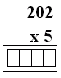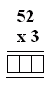Name: ___________________Date:___________________

 Email us to get an instant 20% discount on highly effective K-12 Math & English kwizNET Programs!

### Grade 3 - Mathematics9.1 Multiplication Pictures

 Method: When multiplying two numbers, consider the first number. The first number is multiplied by each of the digits of the second number. Add the products. Example: 12 x 13 = ?Multiply 12 and 3(always start with ones). Multiply 12 and 1. (this is shifted one place to left) Add the products. Answer: 156 Directions: Multiply the following. Also write at least ten examples of your own.
 Q 1: Find the product of the multiplication.102010001010 Q 2: Find the product of the multiplication.556566 Q 3: Find the product of the multiplication.156160155 Q 4: Find the product of the multiplication.420242304200 Question 5: This question is available to subscribers only! Question 6: This question is available to subscribers only!

#### Subscription to kwizNET Learning System costs less than \$1 per month & offers the following benefits:

• Unrestricted access to grade appropriate lessons, quizzes, & printable worksheets
• Instant scoring of online quizzes
• Progress tracking and award certificates to keep your student motivated
• Unlimited practice with auto-generated 'WIZ MATH' quizzes